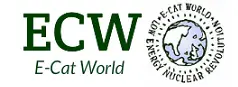The following post has been submitted by Carl-Oscar Gullström, a doctoral student at the Uppsala University, Sweden

Recently there have been some post about LENR thoery using p+Li7->2 He4 as the main source of energy. I just want to point out that even if the reaction generates low radiaton it would at the same time have radiation levels in several order of magnitide above background level. Assuming that a reaction produces 1 MeV of energy LENR experiments where radiaton is below background level while producing energies has an average of 10^12 reactions per second. To compare with an average background level of radiation one can set up a very average gamma rate per second for measurement of radiation done at 0,5 m away depending on gamma energy:

E(gamma)=10 keV 10^6

E(gamma)=100 keV 10^5

E(gamma)=1 MeV 10^4

All previous measuremnt of low energy protons fusion with lithium has Be8 going into an exited state at that is least 300 keV below p+Li thresh hold so the reaction is really p+7Li->8Be+gamma. This would generate 10^12 ~100 kev gammas per second ie a radiation ten million times larger than the background level.

Even if there where a hidden state in 8Be that lies exactly at the p+7Li energy and had 100% alpha decay the alpha energy is to high. Normal alpha radiation from heavy element is about 1 MeV but here the kinetic energy of the alpha particles are about 9 MeV. The result is that the energy is high enough for the alpha particles to interact with other nucleons. For example Nickel+9 MeV alpha would create radioactive copper at a probabilty rate of 10^-4 per released alpha. Radioactive copper generates gamma photons in the MeV range so radiation would still be about a million times to high. It is nothing that one would reccomend to carry around in your pocket.

What is possible from a radiation point of wiev is beta minus unstable nucleids without secondary gamma ie creation of for example 3H, 6He and 63Ni. The high energy electrons from beta minus decay does not interact with the nucleons(at the same rate) and the only secondary radiation is x-ray from interaction with which has an reduction factor about 10^-6 ie in the allowed range. Also possible if one demands alpha decay is to have nuclear transmutation of a bound proton into Lithium. There is strong evidence that Mn+Al->Cr+Si have takend place then one could also think about reactions Mn+Li7->Cr+8Be*->Cr+2Alpha (10MeV). The probability that the alphas would react with surrounding nucleids is exponential reduced with energy so at 5 MeV it is neglectible compared to 9 MeV.

For people that want to work with LENR theories there are two major problems: The interaction range of the strong force and the absence of gamma radiation.

Other experimental observations that could be related is the proton radius puzzle (the proton has a different radius when measured with muons), no electricdipole moment in nucleons (which is not forbidden by the quark-gluon model) and the extra baryons observed in the universe (the matter-antimatter asymmetry).

The theory behind the radius of the proton and neutron is not sufficient to rule out that low energy at zero distance spin dependent electron-nucleon interaction could increase the radius of the nucleons.

The absence of an electric dipole moment in nucleons is an indication on why there is no gamma in LENR. Photons have a dipole moment so if there where a dipole moment in baryons then it could be a decay where a photon is present so the lightest baryon is the one with no dipole moment. At the same time there is an internal structure with charges in the baryons then there must exist a force stronger than the electromagnetic force that could hold two photons in exact opposite direction still. As long as one conserves the no electic dipole law there is nothing that forbids that low energy at zero distance spin dependent electron-nucleon interaction could increase the radius of the proton and neutron.

Carl-Oscar Gullström